# Setup for 2 Player a Game of Food Chain Magnate

Originally generated on 10/11/2018 8:12:44 PM
A direct link to this setup is https://www.BoardgameHelpers.com/FoodChainMagnate/LoadGame.aspx?id=\$2a\$04\$71HubBL9fJcv36pJqk5xau

## Player Setup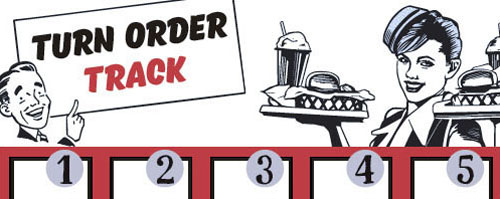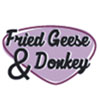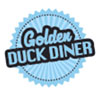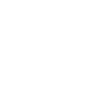## Other Setup

Bank starts with \$100.00
Remove these Billboards: #12, #15, #16
Number of 1x Employee cards used = 1

## Map (Dehydration Land)

Click here to view Map Tile Lettering Key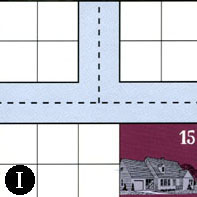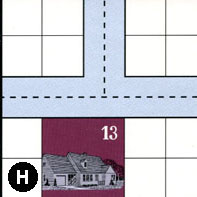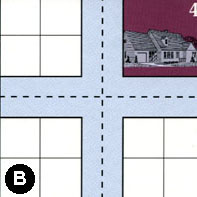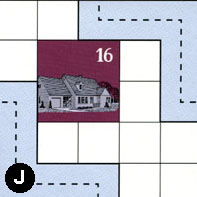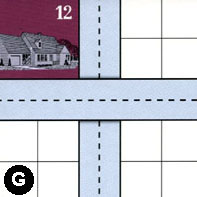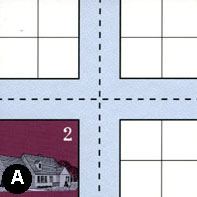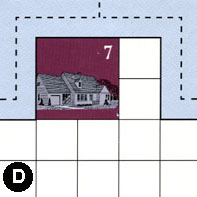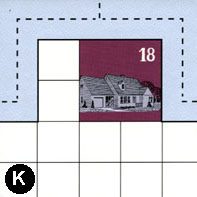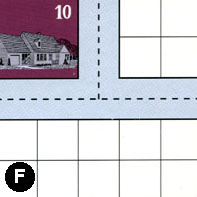### Map Stats

Map Option = Dehydration Land
Total Number of Tiles = 9
Total Number of Starting Houses = 9
Total Number of Beer Spots = 0
Total Number of Soda Spots = 0
Total Number of Lemonade Spots = 0
Total Number of Drink Spots = 0
Number of independent path systems = 1
Number of independent neighborhoods = 9

Path System 1
Size = Large
Number of Tiles = 9
Number of Paths = 31
Contains Loop(s) = No
Number of Starting Houses = 9
Starting Houses Pct of Map (%) = 100
Number of Beer Spots = 0
Number of Soda Spots = 0
Number of Lemonade Spots = 0
Number of Drink Spots = 0
Drink Spots Pct of Map (%) = NaN
Tile Ids = A, B, D, F, G, H, I, J, K
Paths = IWE, JWS, GNS, AWS, BWE, BWN, BNE, AWN, ANE, FWN, KWE, DWE, DWN, JEN, DNE, KWN, GWE, HWN, HNE, HWE, KNE, FWE, FNE, AWE, BES, BWS, BNS, AES, ANS, IWN, INE What do these mean?
Starting House Ids = 2, 4, 7, 10, 12, 13, 15, 16, 18

Neighborhood 1
Total Size = Small
Number of Total Spaces = 4
Number of Empty Spaces (for new houses & gardens) = 4
Number of Beer Spots = 0
Number of Soda Spots = 0
Number of Lemonade Spots = 0
Number of Drink Spots = 0
Number of Starting Houses = 0
Starting Houses Pct of Map (%) = 0
Tile Ids = I
Neighborhood 2
Total Size = Medium
Number of Total Spaces = 22
Number of Empty Spaces (for new houses & gardens) = 18
Number of Beer Spots = 0
Number of Soda Spots = 0
Number of Lemonade Spots = 0
Number of Drink Spots = 0
Number of Starting Houses = 1
Starting Houses Pct of Map (%) = 11
Tile Ids = G, H, I
Starting House Ids = 15
Neighborhood 3
Total Size = Large
Number of Total Spaces = 26
Number of Empty Spaces (for new houses & gardens) = 18
Number of Beer Spots = 0
Number of Soda Spots = 0
Number of Lemonade Spots = 0
Number of Drink Spots = 0
Number of Starting Houses = 2
Starting Houses Pct of Map (%) = 22
Tile Ids = A, B, G, H
Starting House Ids = 2, 13
Neighborhood 4
Total Size = Small
Number of Total Spaces = 4
Number of Empty Spaces (for new houses & gardens) = 4
Number of Beer Spots = 0
Number of Soda Spots = 0
Number of Lemonade Spots = 0
Number of Drink Spots = 0
Number of Starting Houses = 0
Starting Houses Pct of Map (%) = 0
Tile Ids = B
Neighborhood 5
Total Size = Medium
Number of Total Spaces = 23
Number of Empty Spaces (for new houses & gardens) = 15
Number of Beer Spots = 0
Number of Soda Spots = 0
Number of Lemonade Spots = 0
Number of Drink Spots = 0
Number of Starting Houses = 2
Starting Houses Pct of Map (%) = 22
Tile Ids = G, I, J
Starting House Ids = 12, 16
Neighborhood 6
Total Size = Medium
Number of Total Spaces = 8
Number of Empty Spaces (for new houses & gardens) = 4
Number of Beer Spots = 0
Number of Soda Spots = 0
Number of Lemonade Spots = 0
Number of Drink Spots = 0
Number of Starting Houses = 1
Starting Houses Pct of Map (%) = 11
Tile Ids = A, B
Starting House Ids = 4
Neighborhood 7
Total Size = Medium
Number of Total Spaces = 12
Number of Empty Spaces (for new houses & gardens) = 8
Number of Beer Spots = 0
Number of Soda Spots = 0
Number of Lemonade Spots = 0
Number of Drink Spots = 0
Number of Starting Houses = 1
Starting Houses Pct of Map (%) = 11
Tile Ids = A, F, G
Starting House Ids = 10
Neighborhood 8
Total Size = Medium
Number of Total Spaces = 8
Number of Empty Spaces (for new houses & gardens) = 8
Number of Beer Spots = 0
Number of Soda Spots = 0
Number of Lemonade Spots = 0
Number of Drink Spots = 0
Number of Starting Houses = 0
Starting Houses Pct of Map (%) = 0
Tile Ids = A, F
Neighborhood 9
Total Size = Large
Number of Total Spaces = 42
Number of Empty Spaces (for new houses & gardens) = 34
Number of Beer Spots = 0
Number of Soda Spots = 0
Number of Lemonade Spots = 0
Number of Drink Spots = 0
Number of Starting Houses = 2
Starting Houses Pct of Map (%) = 22
Tile Ids = D, F, K
Starting House Ids = 7, 18

v2.1.5